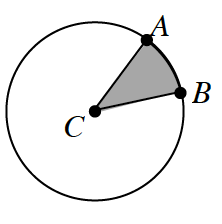### Home > CCG > Chapter Ch10 > Lesson 10.1.4 > Problem10-45

10-45.

The spinner at right is designed so that if you randomly spin the spinner and land in the shaded sector, you win \$$1,000,000$. Unfortunately, if you land in the unshaded sector, you win nothing. Assume point $C$ is the center of the spinner.1. If $m∠ACB = 90º$, how many times would you have to spin to reasonably expect to land in the shaded sector? How did you get your answer?

$4$ times
2. What if $m∠ACB = 1º$? How many times would you have to spin to reasonably expect to land in the shaded sector?
3. Suppose $P(\text{winning}\space 1,000,000) = \frac { 1 } { 5 }$ for each spin. What must $m∠ACB$ equal? Show how you got your answer.
$\frac{360º}{5}=72º$